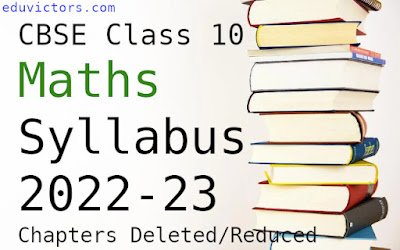# CLASS 10 2022-23 MATHS Detailed Syllabus

CH-1: Real Numbers

Deleted- Euclid’s Division Lemma (Eg- 2,3) & (Exercise 1.1- Q 2,4,5)

CH-2: Polynomials

Deleted- Division Algorithm for Polynomials.

Pg 33 onwards deleted & Exercise- 2.3 deleted

CH-3: Pair of Linear Equations in 2 Variables

Deleted- Cross Multiplication Method

Don’t use this method in solving the questions

Deleted- Completing the square method

CH-5: Arithmetic Progression

No Reduction

CH-6: Coordinate Geometry

Deleted- Area of Triangle

CH-7: Triangles

Deleted-

1. (Motivate) If a perpendicular is drawn from the vertex of the right angle of a right

triangle to the hypotenuse, the triangles on each side of the perpendicular are similar

to the whole triangle and to each other.

2. (Prove) The ratio of the areas of two similar triangles is equal to the ratio of the

squares of their corresponding sides.

3. (Prove) In a right triangle, the square on the hypotenuse is equal to the sum of the

squares on the other two sides.(Pythagoras Theorem)

4. (Prove) In a triangle, if the square on one side is equal to the sum of the squares on the other two sides, the angle opposite to the first side is a right angle.(Converse of Pythagoras Theorem)

In Short- Exercise 6.4 and 6.5 Not in syllabus.

CH-8: Introduction to Trigonometry

No Reduction

CH-9: Some Applications of Trigonometry

No Reduction

CH-10: Circles

No Reduction

CH-11: Constructions

Full Chapter Deleted

CH-12: Area Related to Circles

No Reduction

CH-13: Surface Areas and Volume

Deleted- Frustum of Cone (i.e. Ex 13.4)

CH-14: Statistics

Deleted- Ogive (i.e. Ex 14.4)

CH-15: Probability

No Reduction